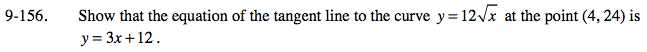### Home > PCT > Chapter Ch9 > Lesson 9.3.5 > Problem9-156

9-156.Use the point - slope form for a linear function.
You are given a point. So now you need the slope of the tangent line.

1. Find the slope function at x = 4.
2. Graph the slope function.
3. As h→0, what does the slope approach?

$\lim_{ h \to 0}\frac{12\sqrt{4+h}-12\sqrt{4}}{h}$

Use the slider in the eTool below to see that the slope at x = 4 is 3. Then find the equation of the tangent line with the given point and slope.
Click the link at right for the full version of the eTool: PCT 9-156 HW eTool